Author: Oscar Cronquist Article last updated on November 15, 2017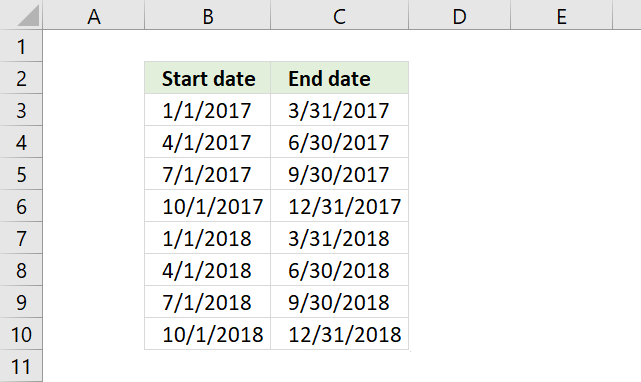I will demonstrate three different methods to build quarterly date ranges in this article.

The two first methods have a date in a cell each where the third method has two dates in a cell.

### Create a quarterly date range using a clever built-in feature in Excel

Excel lets you copy formulas and data using the fill handler, a handy tool that automates your work. It is also smart enough to create number sequences.

Conveniently Excel dates are in fact numbers so the fill handler works fine with dates, as well.

Type the start and end date of the first quarterly date range in row 3. Type the second date range in row 4, see picture below.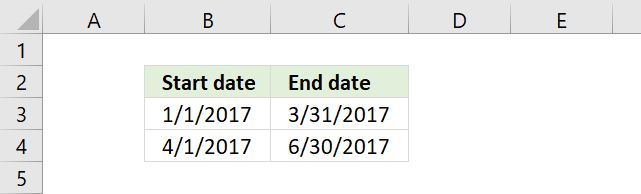Select cell range B3:C4. Press and hold on the black dot.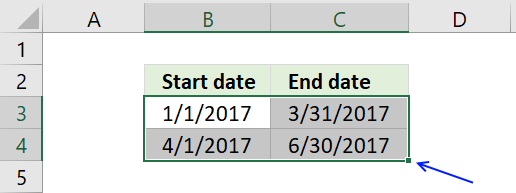Drag to cells below as far as needed.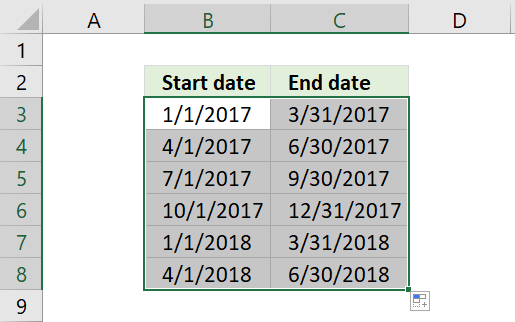Excel automatically creates the following date ranges using the selected cells as a guide to determine the range and starting point.

### Quarterly date ranges using a formula

You also have the option to build a date range using formulas. I have entered 1/1/2017 in cell B3 and the following formula in cell C3:

=DATE(YEAR(B3),MONTH(B3)+3,1)-1

See picture below. The formula adds 3 months to the date in cell B3 and then subtracts 1 to get the last date for the first quarterly date range. This setup takes into account that months have 30 or 31 days.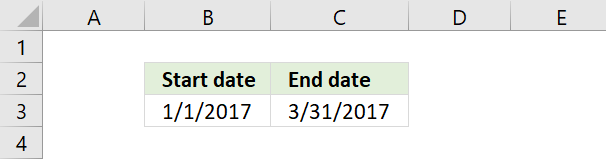Type this formula in cell B4:

=DATE(YEAR(B3),MONTH(B3)+3,1)

It is almost identical to the formula in cell C3. Then copy cell C3 and paste to cell C4.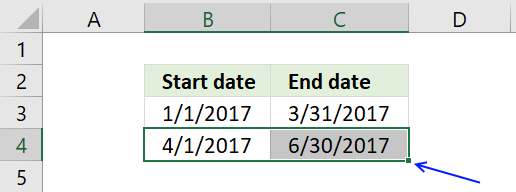Now select cell range B4:C4 and press and hold on black dot, see picture above.

Drag to cells below as far as needed. This action copies the formulas in cell range B4:C4 and pastes them to cells below.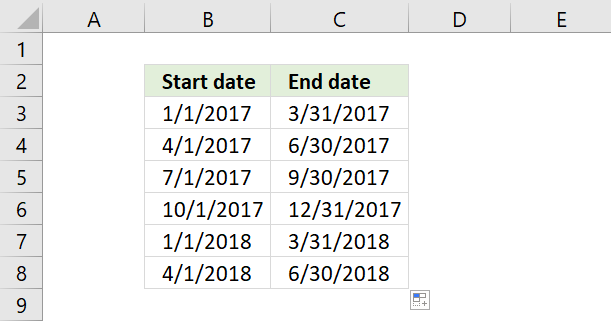### Date ranges in one cell each

The following picture shows date ranges in a cell each, to achieve that we need to build a somewhat more complicated formula. The formulas in cell A4 and A7 must be copied to cells to the right of the start cell.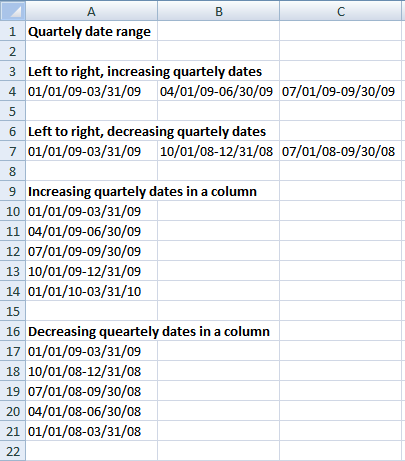### Array formula in A4:

=TEXT(DATE(2009, IF(COLUMN(A:A)=1, 1, COLUMN(A:A)*3-2),  1),  "MM/DD/YY")&"-"&TEXT(DATE(2009, COLUMN(A:A)*3+1,  1)-1,  "MM/DD/YY") + ENTER

copied right as far as necessary.

### Array formula in A7:

=TEXT(DATE(2009, IF(COLUMN(A:A)=1, 1, 1-(COLUMN(A:A)-1)*3),  1),  "MM/DD/YY")&"-"&TEXT(DATE(2009, IF(COLUMN(A:A)=1, 4, 0-(COLUMN(A:A)-2)*3+1),  1)-1,  "MM/DD/YY") + ENTER

copied right as far as necessary.

### Array formula in A10:

=TEXT(DATE(2009, IF(ROW(1:1)=1, 1, ROW(1:1)*3-2),  1),  "MM/DD/YY")&"-"&TEXT(DATE(2009, ROW(1:1)*3+1,  1)-1,  "MM/DD/YY") + ENTER

copied down as far as necessary.

### Array formula in A17:

=TEXT(DATE(2009, IF(ROW(1:1)=1, 1, 1-(ROW(1:1)-1)*3),  1),  "MM/DD/YY")&"-"&TEXT(DATE(2009, IF(ROW(1:1)=1, 4, 0-(ROW(1:1)-2)*3+1),  1)-1,  "MM/DD/YY") + ENTER

copied down as far as necessary.